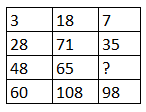Question 13

# Study the given pattern carefully and select the number that can replace the question mark (?)in it.Solution

The pattern follows column Rule

$$(48 - 28) \times 3 = 20 t\times 3 = 60$$

$$(71 - 65) \times 18 = 6 \times 18 = 108$$

Next $$(35 - ?) \times 7 = 98$$

$$\Rightarrow 35\times 7 - 7? = 98$$

$$\Rightarrow 245 - 7 ? = 98$$

$$\Rightarrow -7? = 98-245$$

$$\Rightarrow 7 ? = 147$$

$$\Rightarrow ? = 21$$ Ans

• Free SSC Study Material - 18000 Questions
• 230+ SSC previous papers with solutions PDF